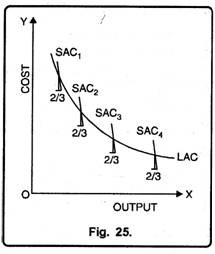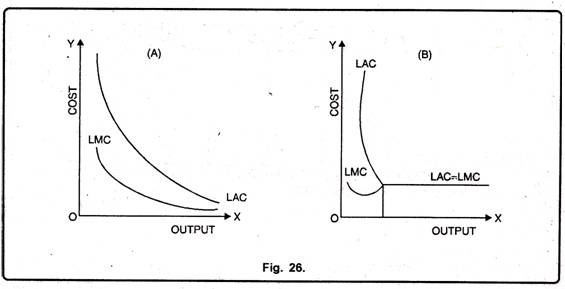# Long Run Average and Marginal Cost Curves

Long Run Average and Marginal Cost Curves!

In the long-run, all costs are variable costs. There is no fixed cost.

According to modern theory of cost curves, long-run average cost curve (LAC) and long-run marginal cost curve (LMC) are L-shaped and not U-shaped as maintained by the traditional theory.

#### Long Run Average Cost Curve:

According to modern theory, long-run costs are mainly of two types:

(1) Production Cost and

(2) Managerial Cost.

On account of increase in production, production cost goes on falling continuously. On the contrary, as the scale of production is enlarged managerial costs may rise. Since fall in production-cost is more than rise in managerial-cost, long-run average cost (LAC) goes on falling with increase in output. In the long-run, each firm makes use of different sizes of plant and equipment.

A given quantity of output needs a particular type of plant relevant to it. Each plant has a short-run average cost (SAC) curve. One can estimate long-run average cost (LAC) with its help. Supposing a firm makes use of four types of plants with different dimensions. Their short-run average cost curves are SAC1, SAC2, SAC3 and SAC4. According to modern theory of cost curves, statistics relating to long-run production reveal that a firm normally makes use of 2/3rd of its plant’s production capacity.It does not make full use of production capacity of its plant. Thus, on the basis of SAC relating to 2/3rd utilization of plant capacity, LAC can be estimated. In Fig. 25 LAC has been shown. It has been obtained by joining together points indicating 2/3rd utilization of production capacity of different short run average cost curves.

#### Long Run Marginal Cost Curves:

According to modern theory, shape of long-run marginal cost curve corresponds to the shape of long-run average cost curve. Relation between LMC curve and LAC curve is shown in Fig. 26 (A) and 26 (B) respectively. Fig. 26 (A) shows that when LAC is L- shaped and LAC curve is falling then LMC curve will also be falling and its falling portion will be below the falling portion of LAC curve.Figure26 (B) shows that when LAC curve is of inverted J- shaped then LMC curve is also of inverted J-shape and remains always below LAC curve. But when LAC curve becomes constant then LMC curve also becomes constant and coincides with it.